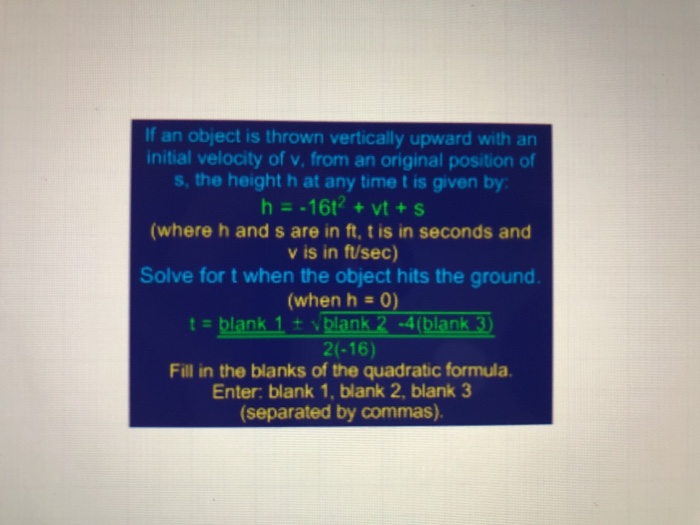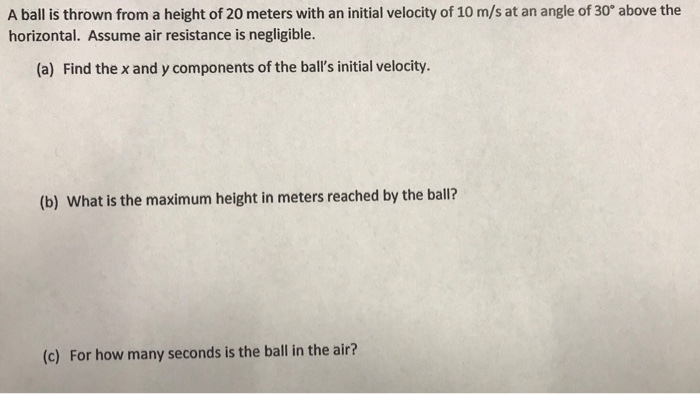Question

# The height(h) of an object that has been dropped or thrown in the air is given by: h(t)=-4.9t^2+vt+h t=time in seconds(s) v=initial velocity in meters per second (m/s) h=initial height in meters(m) A ball is thrown vertically upwardd from the top of the

The height(h) of an object that has been dropped or thrown in the air is given by: h(t)=-4.9t^2+vt+h
t=time in seconds(s)
v=initial velocity in meters per second (m/s)
h=initial height in meters(m)

A ball is thrown vertically upwardd from the top of the Leaning Tower of Pisa (height=53m) with an initial velocity of 30m/s. Find the time(s) at which:
a) the ball's height equals the hight of the tower
b) the ball's height is greater than the height of the tower
c) the ball's height is less than the height of the tower
d)the ball reaches its maximum height

I don't know how to do this problem.

how did you get t=6.12 for a)???
I don't get how you got:
vertex of any parabola is where x = -b/2a = -30/-9.8 = 3.06

h(3.06) = 98.9

where did all the numbers come from?
heck, they gave you the equation.

h(t) = -4.9t^2 + 30t + 53

a) ball goes up, comes back down to the top of the tower. So, we want

53 = -4.9t^2 + 30t + 53
0 = -4.9t^2 + 30t
0 = t(-4.9t + 30)
so, t=0 (at the start) or t = 6.12 (as it comes back down)

b) same calculation but, t is between 0 and 6.12. That is 0 < t < 6.12

c) same calculation, but restricting t to positive values, t>6.12
Naturally, we could also restrict t to the point where height >= 0.

d) vertex of any parabola is where x = -b/2a = -30/-9.8 = 3.06

h(3.06) = 98.9

#### Earn Coins

Coins can be redeemed for fabulous gifts.

Similar Homework Help Questions
• ### The height(H) of an object that has been dropped or thrown in the air is given by: H(t)=-4.9t^2+vt+h t=time in seconds(s) v=initial velocity in meters per second (m/s) h=initial height in meters(m) H=height h=initial height I didn't make this clear

The height(H) of an object that has been dropped or thrown in the air is given by: H(t)=-4.9t^2+vt+h t=time in seconds(s) v=initial velocity in meters per second (m/s) h=initial height in meters(m) H=heighth=initial heightI didn't make this clear on the last post sorry.A ball is thrown vertically upwardd from the top of the Leaning Tower of Pisa (height=53m) with an initial velocity of 30m/s. Find the time(s) at which: a) the ball's height equals the hight of the tower b)...

• ### A launched rocket has an altitude, in meters, given by the polynomial h+vt-4.9t^2, where h is the height, in meters, from which the launch occurs, at the velocity v in meters per second, and the t is the number of seconds for which the rocket is airborne

A launched rocket has an altitude, in meters, given by the polynomial h+vt-4.9t^2, where h is the height, in meters, from which the launch occurs, at the velocity v in meters per second, and the t is the number of seconds for which the rocket is airborne. If a rocket is launched from the top of a tower 150 meters high with an initial upward speed of 60 meters per second, what will its height be after 4 seconds?

• ### I need help with this quadratic equation please: When a ball is thrown, its height in meters h after t seconds is given by the equation h= vt-5t^2 where v is the initial upwards velocity in meters per second

I need help with this quadratic equation please: When a ball is thrown, its height in meters h after t seconds is given by the equation h= vt-5t^2 where v is the initial upwards velocity in meters per second. If meters per second, find all values of t for which h=1 meter. Do not round any intermediate steps. Round the answer to 2 decimal places. My try: Not even sure where to start..

• ### if an object is thrown vertically upward with an initial velocity of v, from an original...if an object is thrown vertically upward with an initial velocity of v, from an original position of s, the height h at any time t is given by: h = -16t^2 + vt + s If an object is thrown vertically upward with an initial velocity of v, from an original position of s, the height h at any time t is given by h16t2 +vt+s (where h and s are in ft, t is in seconds and v...

• ### A ball is thrown from a height of 20 meters with an initial velocity of 10...A ball is thrown from a height of 20 meters with an initial velocity of 10 m/s at an angle of 30° above the horizontal. Assume air resistance is negligible. (a) Find the x and y components of the ball's initial velocity. (b) What is the maximum height in meters reached by the ball? (c) For how many seconds is the ball in the air?

• ### The height of a ball thrown vertically upward from a rooftop is modelled by h(t)=-5t^2=20t=50, where h(t) is the ball's height above the gorund,in meters, at time t seconds after the throw a) determine the maximum height of the ball b) how long does it

The height of a ball thrown vertically upward from a rooftop is modelled by h(t)=-5t^2=20t=50, where h(t) is the ball's height above the gorund,in meters, at time t seconds after the throwa) determine the maximum height of the ballb) how long does it take for the ball to reach its maximum height c) how highis the rooftop

• ### A stone thrown downward with an initial velocity of 34.3 m/sec travel a distance of s meters, where: s(t)=4.9t + 34.3t and t is in seconds

A stone thrown downward with an initial velocity of 34.3 m/sec travel a distance of s meters, where:s(t)=4.9t + 34.3t and t is in seconds. If a stone is thrown downward at 34.3m/sec from a height of 294 m, how long will it take the stone to reach the ground?I am putting 294 in for t and trying to solve it that way. Is this correct? If not please let know where I am going wrong.

• ### on earth, in the absence of air, the rock in excersise 13 would reach a height of s=24t- 4.9t^2 meters in t seconds

on earth, in the absence of air, the rock in excersise 13 would reach a height of s=24t- 4.9t^2 meters in t seconds. How high would the rock go?(exercise 13:a rock thrown vertically upward from the surface of the moon at a velocity of 24m/sec reaches aheight of s=24t-0.t^2.

• ### An object thrown vertically upward from the surface of a celestial body at a velocity of 24 m/s reaches a height of s = -0.6t2 + 24t meters in t seconds.

An object thrown vertically upward from the surface of a celestial body at a velocity of 24 m/s reaches a height of s = -0.6t2 + 24t meters in t seconds. a. Determine the velocity v of the object after t seconds. b. When does the object reach its highest point? c. What is the height of the object at the highest point? d. When does the object strike the ground? e. With what velocity does the object strike the ground? f. On what intervals is...

• ### A 3.00 kg ball is thrown vertically into the air with an initial velocity of 15.0 m/s

A 3.00 kg ball is thrown vertically into the air with an initial velocity of 15.0 m/s. The maximum height of the ball is